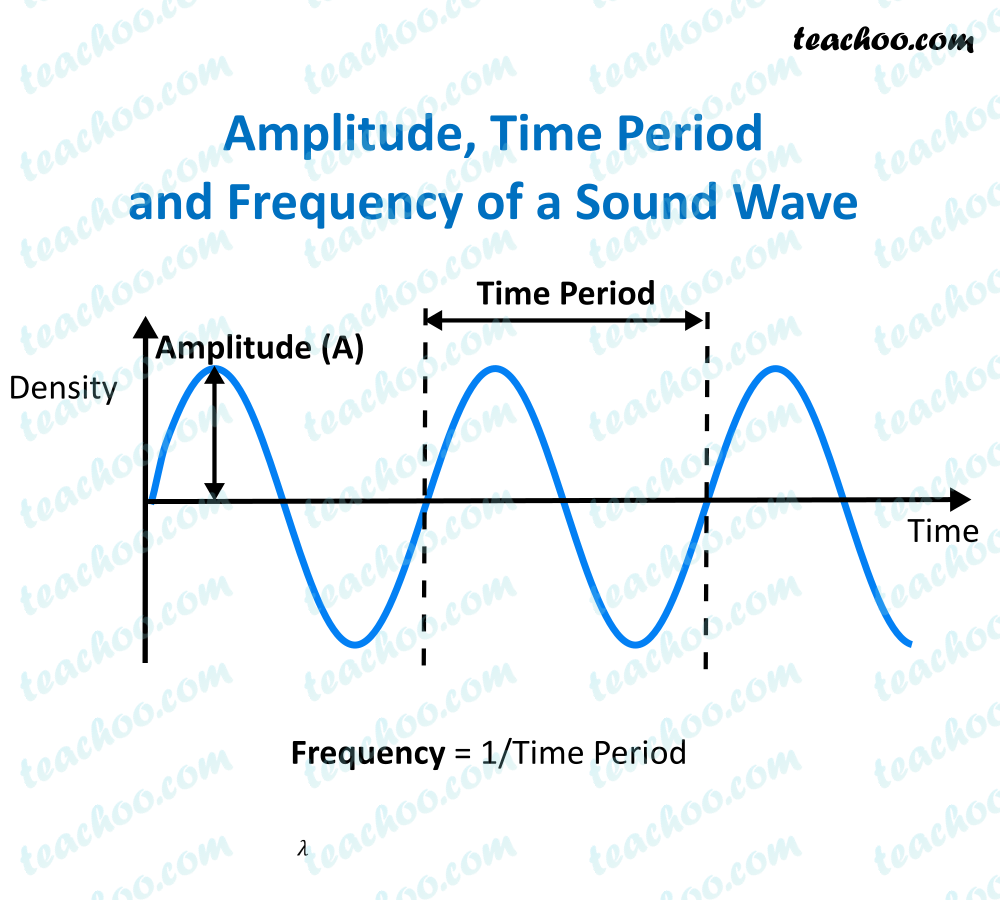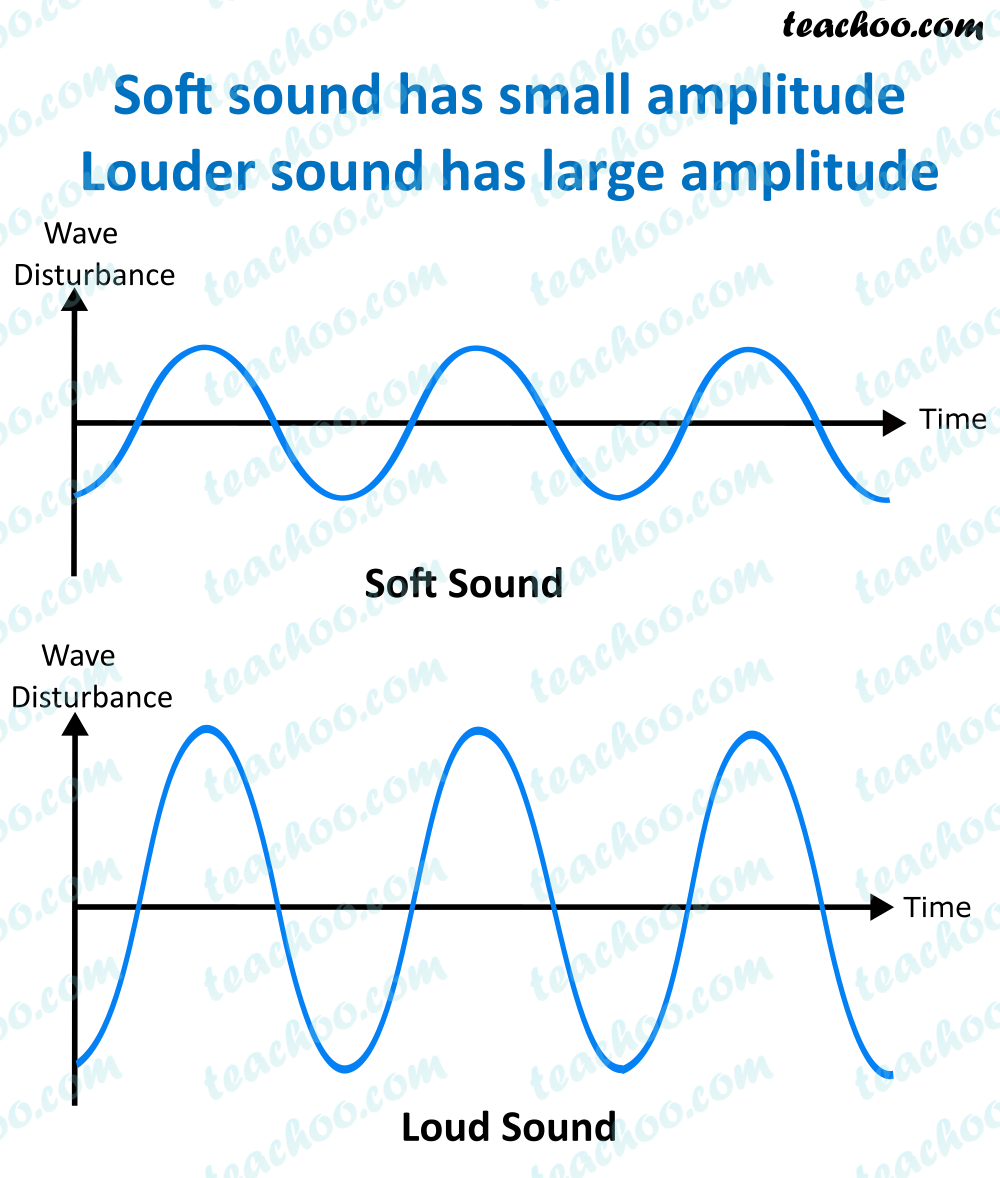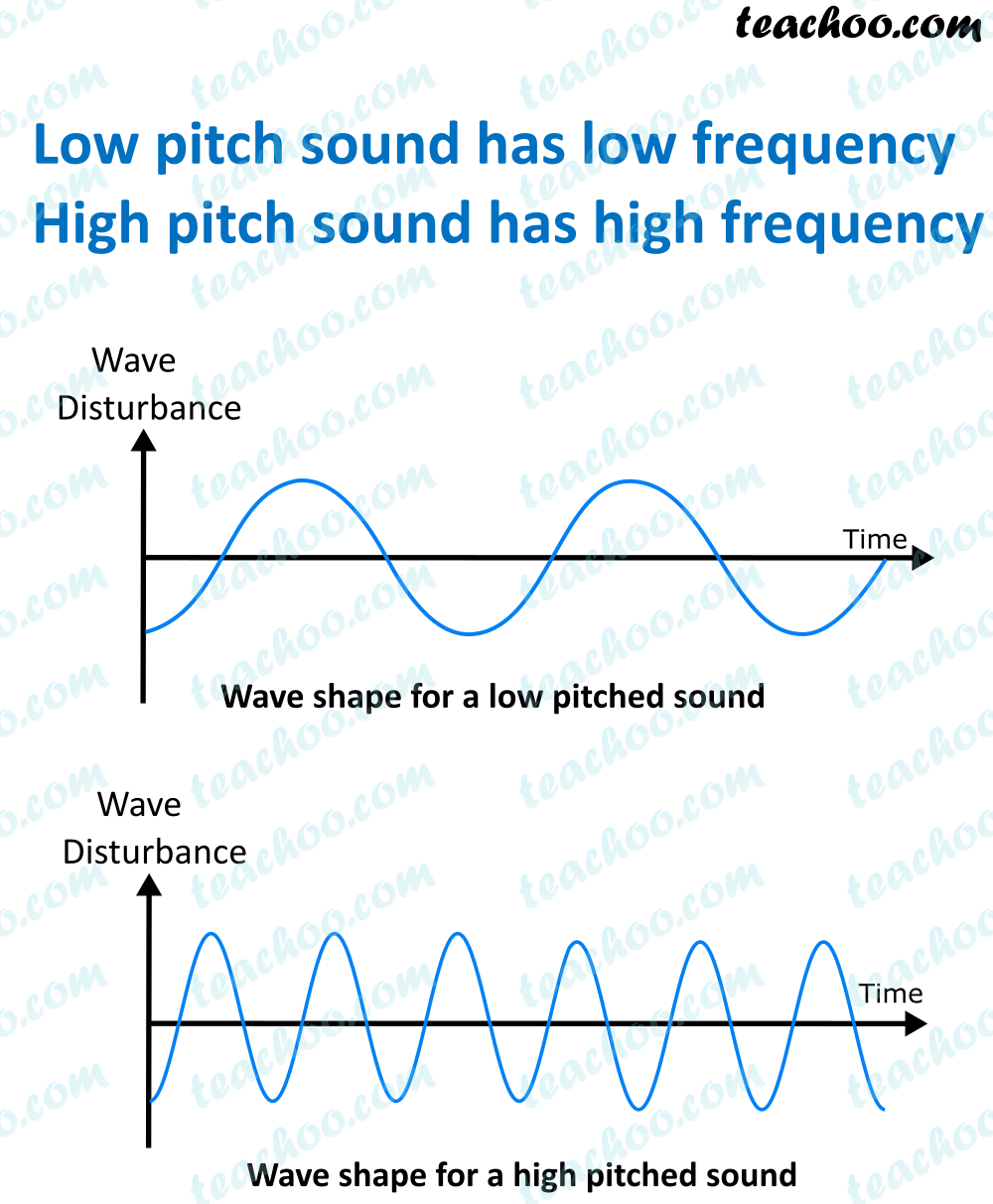Concepts

Class 9
Chapter 12 Class 9 - Sound

Sound Wave is characterized by

• Frequency
• Amplitude
• Wavelength
• Time period
• SpeedLet's study these one by one

## What is Amplitude

It is maximum displacement of a vibrating object from its central location

Explanation

We know that sound is produced due to vibration of particles (To and Fro Motion)

In to and fro motion, an object moves from its central position

More it moves from its central position, more is the amplitude

Less it moves from its central position, less is the amplitude

Note - More Amplitude means More Loudness of Sound

Loudness is directly proportional to the square of Amplitude

If amplitude becomes double, loudness becomes 4 times

If amplitude becomes half,loudness becomes ¼ times

Note:- Roaring of lion can be heard over long distances because it has higher amplitudeBefore we study frequency, lets learn what frequency means

## What is one complete oscillation?

The change in density from the maximum value to the minimum value , then again to the maximum value makes one complete oscillation.

## Time Period of Sound

The time taken by the wave for one complete oscillation is called the time period

It is denoted by t.

It's SI unit is second (s)

## Frequency of Sound

The number of oscillations made in one second is called the frequency of the sound wave.

It is denoted by nu (ν)

Its SI unit is Hertz (Hz)

Explanation

We know that sound is produced due to vibration of particles(To and Fro Motion)

More times it moves in one second,more is the frequency

Less times it moves in one second,less is frequency

Example 1

Suppose if vibration of sound is 50 vibrations per second, it means its frequency is 50 hz

Note - Frequency determines shrillness or pitch of sound

More the frequency, more the shrillness of sound

Less the frequency, less the shrillness of sound

Example 1

• Voice of Baby is of high pitch
• Voice of grown up man is low pitch

Example 2

• Chirping of Bird is of high pitch
• Roaring of lion is of low pitch## Relationship between Time Period and Frequency

Time Period = 1/Frequency

Example

Suppose Frequency of an Object is 20 Hertz

It means that it makes 20 vibrations in 1 second

Time taken for 1 vibration=1/20=0.05 seconds

Check it here

Check it here

## Questions

Question 1 Page 166 (Right Top) - What are wavelength, frequency, time period and amplitude of a sound wave?

Learn in your speed, with individual attention - Teachoo Maths 1-on-1 Class